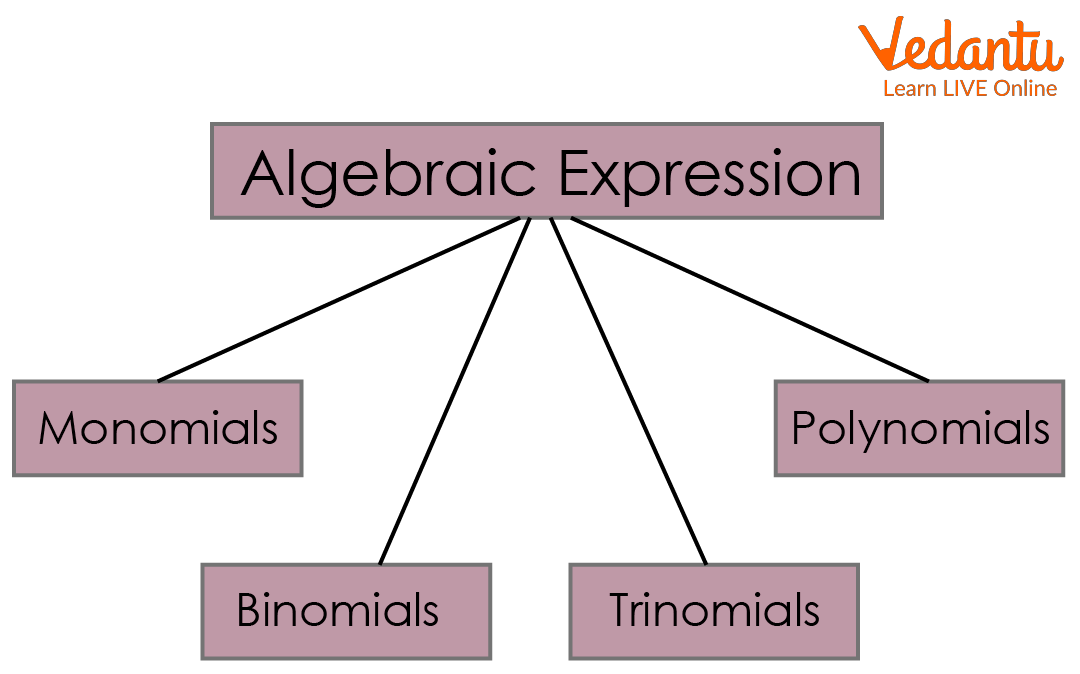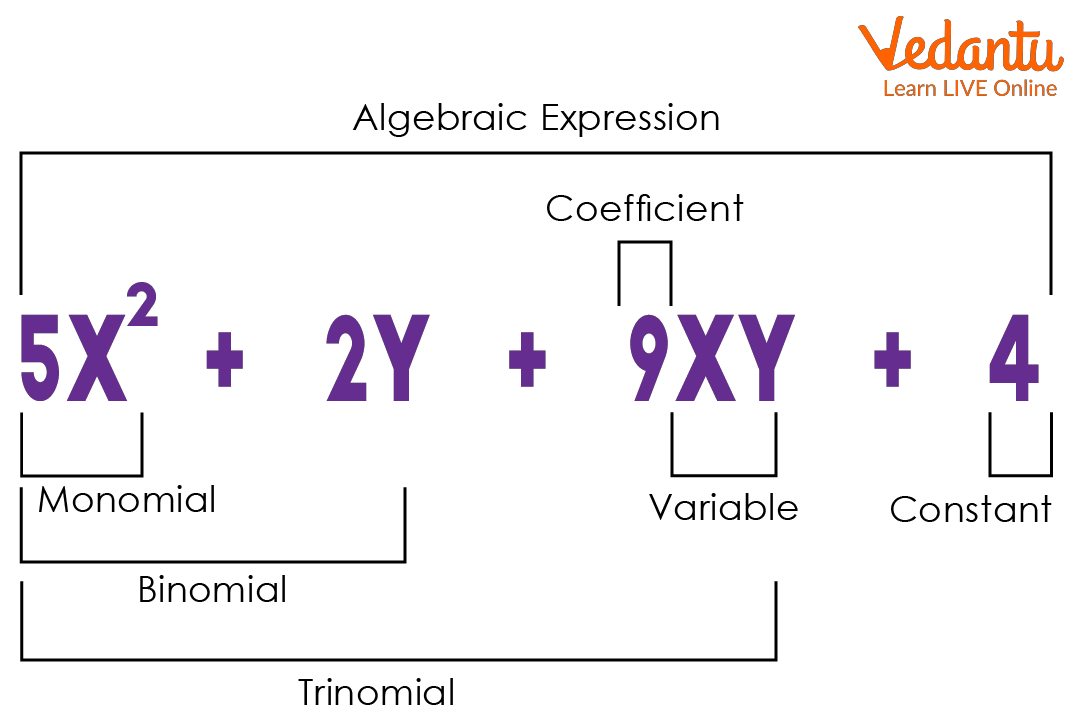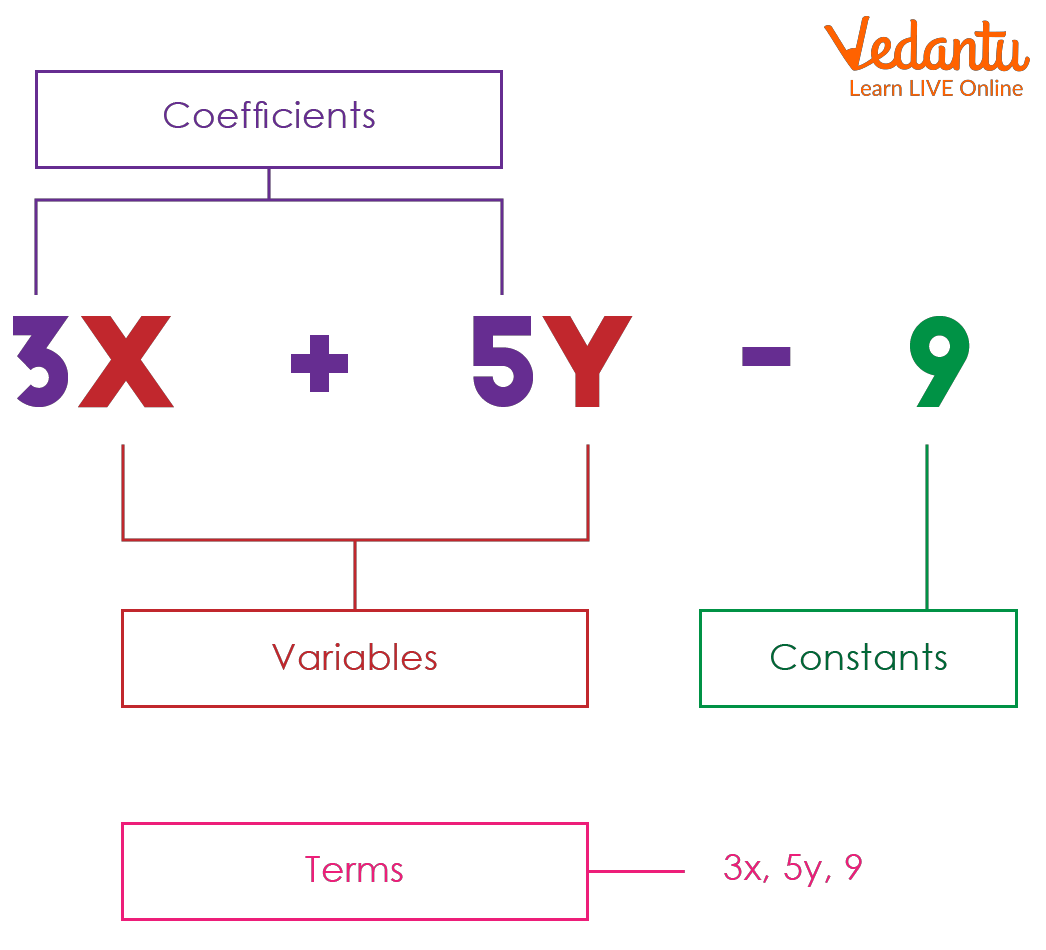Courses
Courses for Kids
Free study material
Free LIVE classes
More

# Terms in Algebraic ExpressionsLIVE
Join Vedantu’s FREE Mastercalss

An algebraic expression is a mathematical expression consisting of variables and constants using mathematical operations such as multiplication, division, addition, and subtraction. It is made up of terms like "coefficient," "variable," and "constant."

### What is an Algebraic Expression?

An algebraic expression is an expression composed of variables, constants, and algebraic operations such as addition, subtraction, division, and multiplication.

For e.g. $2{\rm{x}} + 3 = 0$

Algebraic expression is composed of terms , coefficient, variable and constant.

Few more examples:

• $4x$

• $2a + 3b$

• $3{x^2} - 4xy + 2{y^2}^{}$

### Types of Algebraic Expressions

There are three main types of algebraic expressions that contain:Types of Algebraic Expression

• Monomial : An algebraic expression with only one term is called a monomial.

Examples 3x, 9y, and so on.

• A binomial expression is an algebraic expression made up of two different terms.

Examples include $5{\rm{x}} + 8{\rm{y}}$, ${\rm{a}} + 4{\rm{b}}$ and so on.

• Trinomial: An algebraic expression made up of three terms is known as trinomial.

Example: $4{\rm{x}} + 3{\rm{y}} + 2{\rm{xy}}$

• Polynomial: An expression with two or more terms with nonnegative integer exponents of a variable is called a polynomial.

Examples $a{\rm{x}} + {\rm{by}} + {\rm{c}}$, $4{\rm{x}} + 4{\rm{y}} + 4{\rm{z}}$ , and so on.Algebraic Expression

### Terms Related to Expression

• Terms: Each expression consists of terms. A term can be a signed number, variable, or constant multiplied by a variable.

• Coefficients in Algebraic Expression: A coefficient is an integer that is either multiplied by the variable it is associated with or written alongside the variable.

• Variable: A variable is defined as a numeric value or literal symbol representing a number whose value keeps on changing.

• Constant in Algebraic Expression: A number whose value cannot be changed.Terms, Coefficient, Variable and Constant

### What is a Term in Algebraic Expression?

Each part of an algebraic expression separated by a plus sign $(+)$ or minus sign $(-)$ is known as a term of the algebraic expression. Division sign $(\div)$ or multiplication sign [ (\times) ] does not separate terms in algebraic expressions. Algebraic expressions can be simple, complex, or constant:

1. A simple algebraic expression consists of a single term.

2. A complex algebraic expression consists of two or more terms.

3. A term in an algebraic expression that has a fixed numerical value and no variables is called a constant term in an algebraic expression.

## Conclusion

In mathematics, an algebraic expression is one that comprises variables, constants, coefficients, and arithmetic operations. The algebraic expression's multiple parts are broken down into the following list. Expressions with a monomial have only one term. The terms in binomial expressions are two. An expression having three terms is referred to as a trinomial, and so on.

## Solved Examples:

1. Define the terms of the following expression.

a]$12{\rm{x}} + 4$

b] $3{\rm{ab}} + 4{\rm{a}} + 2{\rm{abc}}$

Solution:

a] $12x + 4$

Terms: $12x, 4$

b] $3{\rm{ab}} + 4{\rm{a}} + 2{\rm{abc}}$

Terms: $3ab, 4a, 2abc$

1. Classify the types of following algebraic expressions.

1. $12x + 5y$

2. $5{\rm{a}} + 4{\rm{c}} + 5{\rm{ab}}$

Solution:

a]$12x + 5y$

Binomial as it has two terms.

b]$5{\rm{a}} + 4{\rm{c}} + 5{\rm{ab}}$

Trinomial as well as polynomial as it has three term ( more than two terms)

1. Find the value of the expression $5x + 4$ if the value of ${\rm{x}} = - 5$

Solution: $5x + 4$

Substitute ${\rm{x}} = - 5$ in the expression $= 5[ - 5] + 4$

$= - 25 + 4$

$= - 21$

Thus the value of expression is $-21$

1. Is 9 an algebraic expression?

Solution: Yes, 9 is algebraic because it can be viewed as a monomial.

Last updated date: 27th Sep 2023
Total views: 41.7k
Views today: 0.41k

## FAQs on Terms in Algebraic Expressions

1. Is algebraic expression a polynomial?

All algebraic expressions are not polynomials, though. But algebraic expressions are what all polynomials are. The distinction is that algebraic expressions also include irrational numbers in the powers, whereas polynomials only include variables and coefficients with the mathematical operations (+, -, and ).

2. Why are variables and constants important?

Variables and constants play a very important role in mathematics and other subjects. To progress in Maths, the students must know how to read and write math variables and constants. Algebra is considered to be the language of maths, therefore, without knowing the importance and use of algebra, students cannot solve the mathematical problems at higher standards. At the same time, variables and constants are also an important part of computation. Students can visit Vedatu’s study material on variables and constants to learn more about algebraic expressions.

3. Why are algebraic expressions useful?

Algebraic  expressions use variables (using multiple multiples)  to describe real-world scenarios. Instead of "the cost of 3 pens and 4 pencils", you could say $3{\rm{x}} + 4{\rm{y}}$ where x and y are the cost of the pen and pencil, respectively. Also, writing real-world scenarios as expressions helps to perform mathematical calculations.